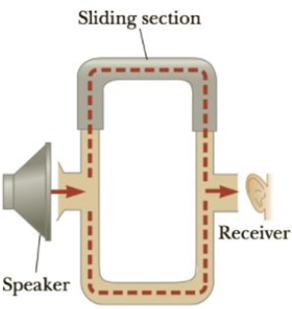Chapter 14, Problem 38P

Chapter
Section
Textbook Problem

The acoustical system shown in Figure P14.38 is driven by a speaker emitting sound of frequency 756 Hz. (a) If constructive interference occurs at a particular instant, by what minimum amount should the path length in the upper U-shaped tube be increased so that destructive interference occurs instead? (b) What minimum increase in the original length of the upper tube will again result in constructive interference?Figure P14.38

(a)

To determine
The increase in distance of path length through the upper arm to produced destructive interference.

Explanation

Given Info: Frequency of the sound from the speaker is 756Hz.

Formula for wavelength,

λ=vf

• v is the velocity of the sound,
• f is the frequency of sound emitted from horn,

The speed of sound is 343m/s.

Substitute 343m/s for v and 756Hz for f in the above expression to get λ

λ=343m/s756Hz=0

(b)

To determine
The minimum increase in the original length of the upper tube will again result in constructive interference.

Still sussing out bartleby?

Check out a sample textbook solution.

See a sample solution

The Solution to Your Study Problems

Bartleby provides explanations to thousands of textbook problems written by our experts, many with advanced degrees!

Get Started

Partially completed proteins are not held for completion at a later time when the diet may improve. T F

Nutrition: Concepts and Controversies - Standalone book (MindTap Course List)

All the human senses have ___. (1.3)

An Introduction to Physical Science

What evidence can you give that granulation is caused by convection?

Horizons: Exploring the Universe (MindTap Course List)